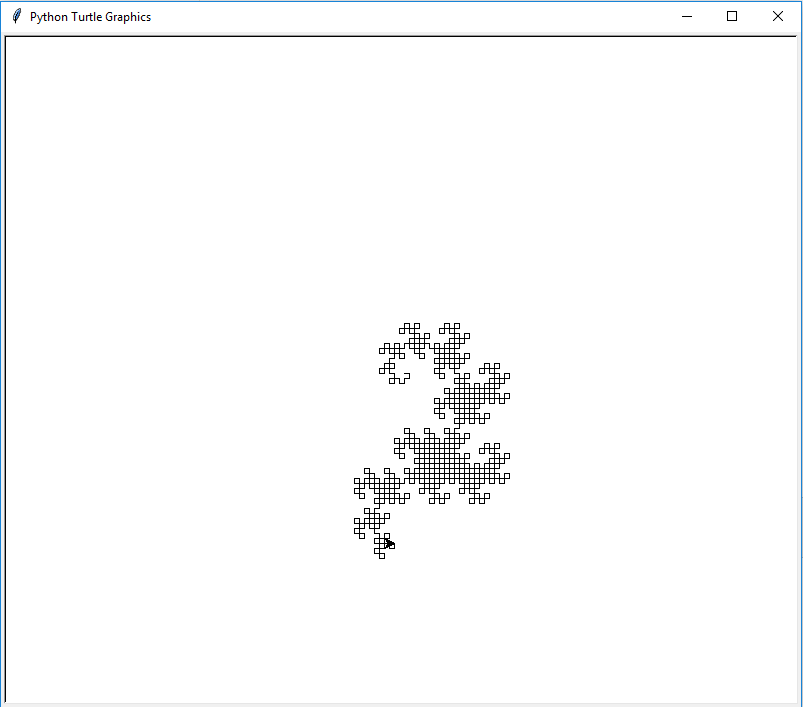# Exercises with 2D computer graphics¶

It is possible to create programming exercises where the student's program creates a picture as an output.

Using mooc-grader inside a Docker container has two restrictions.

First, there is no display inside the grading container. The grading-python-web container is an exception.

Second, mooc-grader gives the grading results and feedback to A+ as HTML document. The HTML document cannot refer to any image files inside the grading container, because that container is shut down before mooc-grader hands the results off to A+. Therefore any pictures that must be shown to student must be encoded into the HTML response using Data URI scheme. In plain English, this means that the image file is converted into text that is placed in the HTML document.

The following exercise is an example on how this is done.

### The turtle exercise¶

The Python programming language has a simple graphics library called turtle. One can use the library with command import turtle. The drawing is analogy of a turtle which moves leaving a line behind. A new turtle is created with command turtle.Turtle(). After that, one can move the turtle with methods forward(), left(), and right().

Example:

import turtle
t = turtle.Turtle()
t.speed(0)          # maximum speed

t.forward(8)
t.right(45)
t.forward(5)
t.left(30)
t.forward(15)


Your task is to draw a pattern with 1000 steps such that the turtle alternatingly moves 5 units forward and then turns 90 degrees either left or right.

The direction of the nth turn is determined with the following method. First divide the number n by 2 until the result is an odd number. Then, if the modulus of that number with four is 1, turn to the right. Otherwise, turn to the left.

A+ presents the exercise submission form here.

1. Create a function get_direction(), which takes the number of the turn and returns the direction, either "right" or "left".

2. One can implement get_direction() for example with a while loop.

3. Notice that in the first turn $$n=1$$.

4. One step is five units forward and then a 90 degree turn.

5. To accelerate the drawing animation, you may use the speed() method of the turtle library.

Here is the result your program should produce.### Commentary on the turtle exercise¶

This is an actual exercise for the Aalto university course CS-A1130 Tietotekniikka sovelluksissa (Applications of computing).

The code for this exercise is found in the directory exercises/turtle_python. Try to submit the file model.py. The another file turtles.py is the code template for the student.

The exercise draws the dragon curve fractal iteratively.

The student uses the real turtle library when testing their solution. The grader software uses another libraries, simpleturtle.py and minipng.py, with minimal functionality to implement the turtle graphics for the exercise and creating a PNG file.

Finally, in minipng.Image.write_to_file() the result is written to a Base64 encoded PNG file. Then Python-grader-utils uses the feedback template feedback_plot_png.html which has the following lines:

<img src="data:image/png;base64,
{% include 'turtles.png.base64' %}
" alt="Graphics" />


That piece of code inserts the Base64 encoded PNG image into the HTML using the Data URI scheme.

Posting submission...